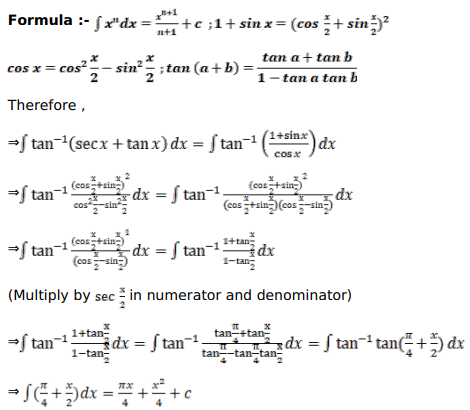# Mark against the correct answer in each of the following:

Question:

Mark $(\sqrt{)}$ ) against the correct answer in each of the following:

$\int \tan ^{-1}(\sec x+\tan x) d x=?$

A. $\frac{\pi x}{4}+\frac{x^{2}}{4}+C$

B. $\frac{\pi x}{4}-\frac{x^{2}}{4}+C$

C. $\frac{1}{\left(1+x^{2}\right)}+C$

D. none of these

Solution: